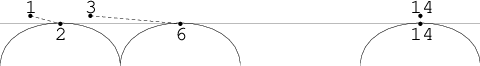Your browser (Unknown 0) is no longer supported. Some parts of the website may not work correctly. Please update your browser.

#### BoatAlignment

Connect N points with N line segments so as to minimize the largest distance between them.

You are helping the port management to organize boats moored in a port. There is a straight wharf with N mooring bollards and N boats. The wharf (and the dock in front of it) is of length M. Each boat has the same width: 2*X. The bollards are located at the very edge of the wharf. It is possible for more than one bollard to be at the same position.

You have to moor each boat to a separate bollard so that the following rules are satisfied:

• each boat is fixed with a single mooring rope to the bank of the wharf,
• the mooring rope connects the middle of the boat's bow with a bollard,
• the middle of the boat's bow can be set only on integer positions,
• the sides of the boats can touch each other,
• boats cannot overlap,
• boats cannot be placed outside the dock or extend it,
• two boats cannot be tied to the same bollard.

All the boats must have mooring ropes of the same length. The goal is to minimize this length.

More formally, let the max distance be the largest distance between the middle of any boat's bow and the bollard to which the boat is moored. The goal is to align the boats so that the max distance is as small as possible.

You are given a non-empty array R of N integers, and two positive integers X and M. Array R contains the positions of the bollards along the wharf. The wharf's ends are at positions 0 and M.

For example, the following array R, and integers X and M:

R = 1 X = 2 R = 3 M = 16 R = 14

describe:

• three bollards at positions 1, 3 and 14,
• three boats of width 4,
• a wharf of length 16.

You can set:

• the center of the first boat at position 2,
• the center of the second boat at position 6,
• the center of the third boat at position 14.Between the first boat and the first bollard the distance is 1; between the second boat and the second bollard it is 3; and between the third boat and the third bollard it is 0; so the max distance is 3.

Write a function:

class Solution { public int solution(int[] R, int X, int M); }

that, given an array R consisting of N integers, given two integers X and M, returns the minimal max distance you can achieve.

If it is not possible to tie all the boats, the function should return −1.

For example, given the following array R, integers X and M:

R = 1 X = 2 R = 3 M = 16 R = 14

the function should return 3, as explained above.

Write an efficient algorithm for the following assumptions:

• N is an integer within the range [1..100,000];
• X and M are integers within the range [1..1,000,000,000];
• each element of array R is an integer within the range [0..M];
• array R is sorted in non-decreasing order.